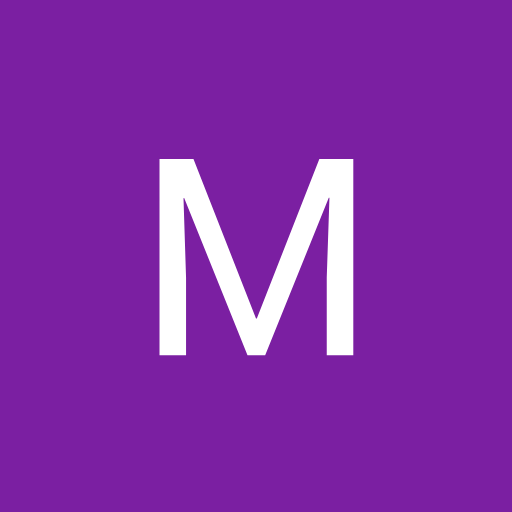# Address 200H contains the byte 3FH. What is the decimal equivalent of 3FH

A. 63

B. 32

C. 16

D. 38

E. None of the above

1.I think this answer us wrong bcs it's a hexadecimal number and we have to multiply echbwith 16^

2.H?

3.3fh
3=11
F=15=1111
3f= (11)(1111) now convert into decimal
111111= 1*2^5+ 1*2^4+ 1*2^3+ 1*2^2+ 1*2^1+ 1*2^0= 32+16+8+4+2+1= 63

4.plz tell me how is ans is 63

Related Questions on Digital Computer Electronics

An OR gate can be imagined as

A. switches connected in series

B. switches connected in parallel

C. MOS transistors connected in series

D. All of the above

E. None of the above